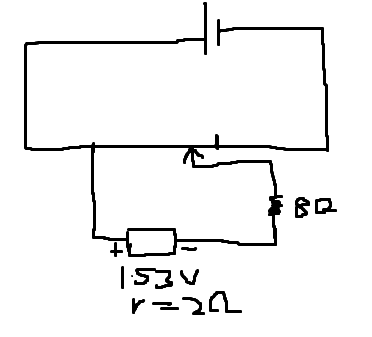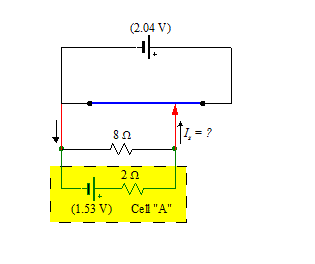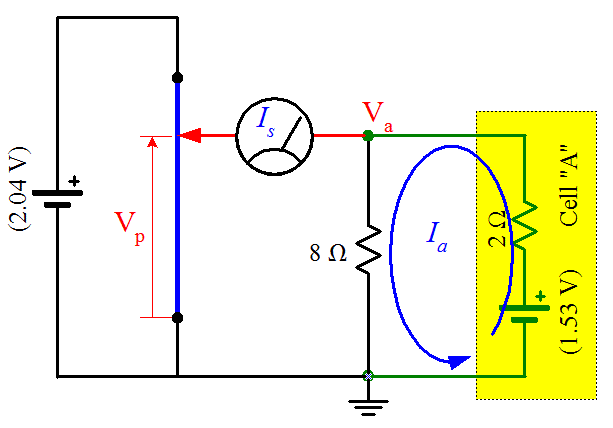# Solving potentiometer problem with addition of resistor

## Homework Statement

The emf of a battery A is balanced by a length of 75 cm on a potentiometer wire. The emf of a standard cell, 1.02V, is balanced by a length of 50 cm. What is the emf of A?
Calculate the new balance length if A has an internal resistance of 2 Ω and a resistor of 8 Ω is joined to its terminals.

E= I(R+r)

## The Attempt at a Solution

I can answer the first part of this question. Emf of A is 1.53 V. It's the second part of the question that I don't know.
With the addition of the 8 Ω resistor, the voltage across the potentiometer wire must be equal to the voltage from A after voltage drop across 8Ω resistor, right?

And if so, I don't know how to find it, for I don't know how to find the value of current. If my reasoning is correct, I will end up with E= I(R+r) + V', where V' is the potential difference across potentiometer wire.

I don't know if I'm totally wrong.

gneill
Mentor

## Homework Statement

The emf of a battery A is balanced by a length of 75 cm on a potentiometer wire. The emf of a standard cell, 1.02V, is balanced by a length of 50 cm. What is the emf of A?
Calculate the new balance length if A has an internal resistance of 2 Ω and a resistor of 8 Ω is joined to its terminals.

E= I(R+r)

## The Attempt at a Solution

I can answer the first part of this question. Emf of A is 1.53 V. It's the second part of the question that I don't know.
With the addition of the 8 Ω resistor, the voltage across the potentiometer wire must be equal to the voltage from A after voltage drop across 8Ω resistor, right?
That depends upon your interpretation of the circuit description. Do you consider the 8 Ω resistor to be in series with the A cell (and its internal resistance of 2 Ω), or in parallel with it? The description does say "terminals" (plural)...

What's the current drawn through the potentiometer connection when the system is balanced? Perhaps sketching your interpretation of the circuit diagram and labeling all known values would be helpful.

That depends upon your interpretation of the circuit description. Do you consider the 8 Ω resistor to be in series with the A cell (and its internal resistance of 2 Ω), or in parallel with it? The description does say "terminals" (plural)...

What's the current drawn through the potentiometer connection when the system is balanced? Perhaps sketching your interpretation of the circuit diagram and labeling all known values would be helpful.Ok, haha this is my interpretation. I don't know how to provide those nice circuits some users here do, so I just use paint. But I think it is clear, I hope.

And it is with this interpretation that I'm stuck. Since you might me wondering what the circuit might be, the answer is 60 cm, if it helps.
And its precisely the current that I don't know how to find.

gneill
Mentor
Okay, so the problem is with your interpretation of the circuit description. The 8 Ω resistor is connected to BOTH terminals of the A cell, not just one. That is, it is in parallel with the cell. See here (I use MS Visio for drawings, by the way):From your knowledge of how a potentiometer circuit works, what is the current ##I_s## when the circuit is balanced?

Okay, so the problem is with your interpretation of the circuit description. The 8 Ω resistor is connected to BOTH terminals of the A cell, not just one. That is, it is in parallel with the cell. See here (I use MS Visio for drawings, by the way):

View attachment 87276

From your knowledge of how a potentiometer circuit works, what is the current ##I_s## when the circuit is balanced?
It's zero. But other than that, I still don't know how to proceed. Is the voltage drop across the potentiometer equal to the voltage across 8 Ω resistor and cell A?
And how did you get 2.04 V?

gneill
Mentor
It's zero. But other than that, I still don't know how to proceed.
If that current is zero then the cell + resistor is essentially an isolated circuit (no current enters or leaves this circuit "island". You can determine the local current and all potential drops in this subcircuit.
Is the voltage drop across the potentiometer equal to the voltage across 8 Ω resistor and cell A?
Yup.
And how did you get 2.04 V?
From the standard cell test conditions described in the problem statement. 50 cm is half the 100 cm of the full potentiometer wire.

•Qwertywerty
If that current is zero then the cell + resistor is essentially an isolated circuit (no current enters or leaves this circuit "island". You can determine the local current and all potential drops in this subcircuit.

Yup.

From the standard cell test conditions described in the problem statement. 50 cm is half the 100 cm of the full potentiometer wire.
Ok, thanks. One last question. Actually, if I put an ammeter at the 8 Ω resistor and at cell A, there would be no reading right? Since there's no net potential difference. You are just isolating the circuit to solve the question right?

Ok, thanks. One last question. Actually, if I put an ammeter at the 8 Ω resistor and at cell A, there would be no reading right? Since there's no net potential difference. You are just isolating the circuit to solve the question right?
No , you would , in both cases .

No , you would , in both cases .
Why? I thought there's no potential difference across resistor and cell A?

You are just isolating the circuit to solve the question right?
We're doing this because there will be current in this loop , which will , however , not move out of this loop ( as galvanometer shows zero reading ) .

We then just write Kirchoff ' s loop law , for this particular loop .

Hope this helps .

Why? I thought there's no potential difference across resistor and cell A?
If there wasn't , then how would the potentiometer work ?

If there wasn't , then how would the potentiometer work ?
I don't understand. I thought that the potential drop across potentiometer is equal to potential drop across resistor and cell A? If so, shouldn't that potential drop be balanced by potential difference across terminals of cell A and resistor? This potential difference is supplied by cell A. Then, how can there be current?

I thought that the potential drop across potentiometer is equal to potential drop across resistor and cell A?
Note : Or ; not And .
If so, shouldn't that potential drop be balanced by potential difference across terminals of cell A and resistor? This potential difference is supplied by cell A. Then, how can there be current?
The previous part of the post should answer this .

gneill
Mentor
It might be beneficial to have a labeled circuit diagram to refer to in order to avoid ambiguities during discussions. Here's an attempt that I hope will help:•Qwertywerty and SammyS